# Measurement of physical optics and microwaves

6 de Nov de 2016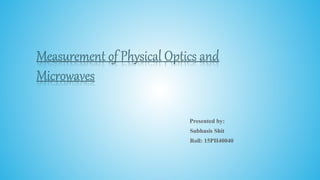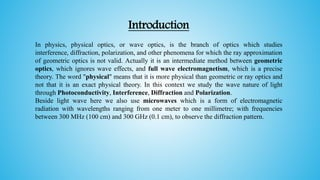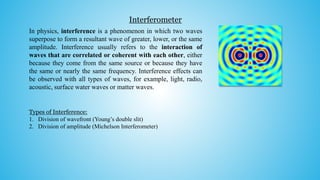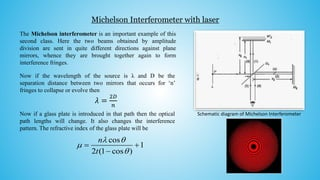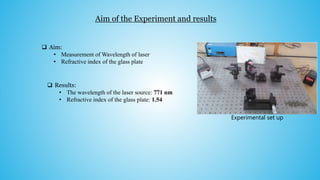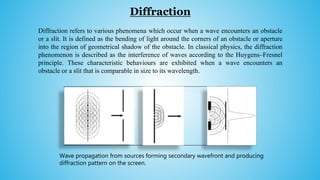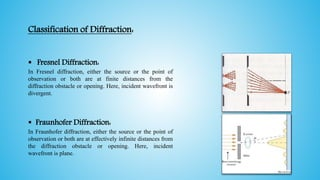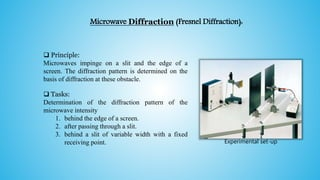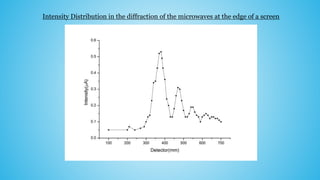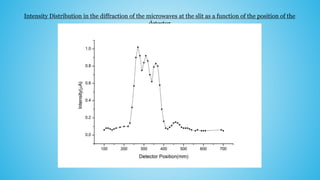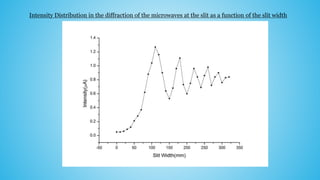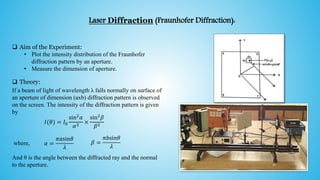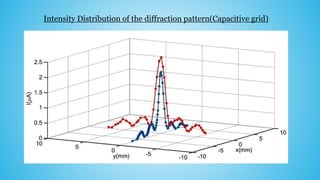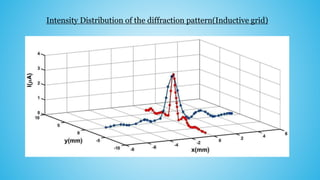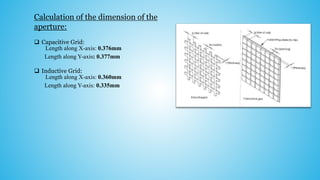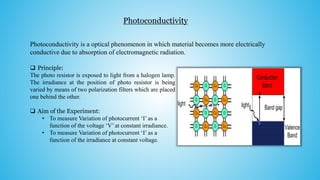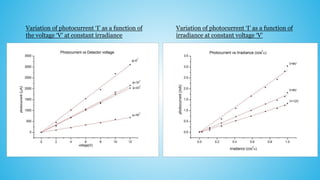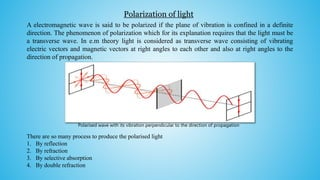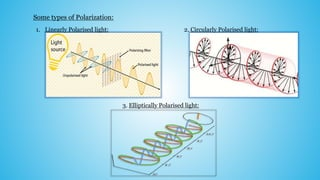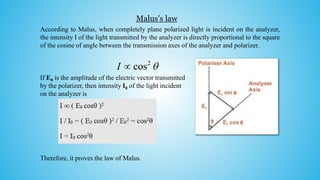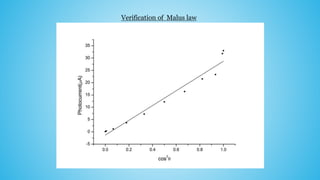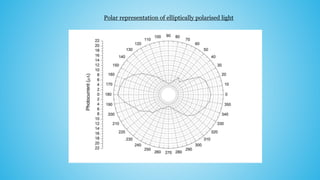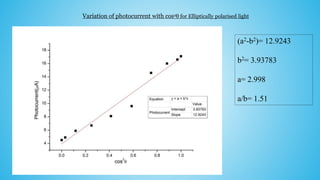1 de 25

### Measurement of physical optics and microwaves

• 1. Measurement of Physical Optics and Microwaves Presented by: Subhasis Shit Roll: 15PH40040
• 2. Introduction In physics, physical optics, or wave optics, is the branch of optics which studies interference, diffraction, polarization, and other phenomena for which the ray approximation of geometric optics is not valid. Actually it is an intermediate method between geometric optics, which ignores wave effects, and full wave electromagnetism, which is a precise theory. The word "physical" means that it is more physical than geometric or ray optics and not that it is an exact physical theory. In this context we study the wave nature of light through Photoconductivity, Interference, Diffraction and Polarization. Beside light wave here we also use microwaves which is a form of electromagnetic radiation with wavelengths ranging from one meter to one millimetre; with frequencies between 300 MHz (100 cm) and 300 GHz (0.1 cm), to observe the diffraction pattern.
• 3. Interferometer In physics, interference is a phenomenon in which two waves superpose to form a resultant wave of greater, lower, or the same amplitude. Interference usually refers to the interaction of waves that are correlated or coherent with each other, either because they come from the same source or because they have the same or nearly the same frequency. Interference effects can be observed with all types of waves, for example, light, radio, acoustic, surface water waves or matter waves. Types of Interference: 1. Division of wavefront (Young’s double slit) 2. Division of amplitude (Michelson Interferometer)
• 4. Michelson Interferometer with laser The Michelson interferometer is an important example of this second class. Here the two beams obtained by amplitude division are sent in quite different directions against plane mirrors, whence they are brought together again to form interference fringes. Now if the wavelength of the source is λ and D be the separation distance between two mirrors that occurs for ‘n’ fringes to collapse or evolve then 𝜆 = 2𝐷 𝑛 Now if a glass plate is introduced in that path then the optical path lengths will change. It also changes the interference pattern. The refractive index of the glass plate will be Schematic diagram of Michelson Interferometer cos 1 2 (1 cos ) n t       
• 5. Aim of the Experiment and results  Aim: • Measurement of Wavelength of laser • Refractive index of the glass plate  Results: • The wavelength of the laser source: 771 nm • Refractive index of the glass plate: 1.54 Experimental set up
• 6. Diffraction Diffraction refers to various phenomena which occur when a wave encounters an obstacle or a slit. It is defined as the bending of light around the corners of an obstacle or aperture into the region of geometrical shadow of the obstacle. In classical physics, the diffraction phenomenon is described as the interference of waves according to the Huygens–Fresnel principle. These characteristic behaviours are exhibited when a wave encounters an obstacle or a slit that is comparable in size to its wavelength. Wave propagation from sources forming secondary wavefront and producing diffraction pattern on the screen.
• 7. Classification of Diffraction:  Fresnel Diffraction: In Fresnel diffraction, either the source or the point of observation or both are at finite distances from the diffraction obstacle or opening. Here, incident wavefront is divergent.  Fraunhofer Diffraction: In Fraunhofer diffraction, either the source or the point of observation or both are at effectively infinite distances from the diffraction obstacle or opening. Here, incident wavefront is plane.
• 8. Microwave Diffraction (Fresnel Diffraction): Experimental set-up  Principle: Microwaves impinge on a slit and the edge of a screen. The diffraction pattern is determined on the basis of diffraction at these obstacle.  Tasks: Determination of the diffraction pattern of the microwave intensity 1. behind the edge of a screen. 2. after passing through a slit. 3. behind a slit of variable width with a fixed receiving point.
• 9. Intensity Distribution in the diffraction of the microwaves at the edge of a screen
• 10. Intensity Distribution in the diffraction of the microwaves at the slit as a function of the position of the detector
• 11. Intensity Distribution in the diffraction of the microwaves at the slit as a function of the slit width
• 12. Laser Diffraction (Fraunhofer Diffraction):  Aim of the Experiment: • Plot the intensity distribution of the Fraunhofer diffraction pattern by an aperture. • Measure the dimension of aperture.  Theory: If a beam of light of wavelength λ falls normally on surface of an aperture of dimension (axb) diffraction pattern is observed on the screen. The intensity of the diffraction pattern is given by where, And θ is the angle between the diffracted ray and the normal to the aperture. 𝐼(𝜃) = 𝐼0 sin2 𝛼 𝛼2 × sin2 𝛽 𝛽2 𝛼 = 𝜋𝑎sin𝜃 𝜆 𝛽 = 𝜋𝑏sin𝜃 𝜆
• 13. Intensity Distribution of the diffraction pattern(Capacitive grid)
• 14. Intensity Distribution of the diffraction pattern(Inductive grid)
• 15. Calculation of the dimension of the aperture:  Capacitive Grid: Length along X-axis: 0.376mm Length along Y-axis: 0.377mm  Inductive Grid: Length along X-axis: 0.360mm Length along Y-axis: 0.335mm
• 16. Photoconductivity  Principle: The photo resistor is exposed to light from a halogen lamp. The irradiance at the position of photo resistor is being varied by means of two polarization filters which are placed one behind the other.  Aim of the Experiment: • To measure Variation of photocurrent ‘I’ as a function of the voltage ‘V’ at constant irradiance. • To measure Variation of photocurrent ‘I’ as a function of the irradiance at constant voltage. Photoconductivity is a optical phenomenon in which material becomes more electrically conductive due to absorption of electromagnetic radiation.
• 17. Variation of photocurrent ‘I’ as a function of the voltage ‘V’ at constant irradiance Variation of photocurrent ‘I’ as a function of irradiance at constant voltage ‘V’
• 18. Polarization of light A electromagnetic wave is said to be polarized if the plane of vibration is confined in a definite direction. The phenomenon of polarization which for its explanation requires that the light must be a transverse wave. In e.m theory light is considered as transverse wave consisting of vibrating electric vectors and magnetic vectors at right angles to each other and also at right angles to the direction of propagation. Polarised wave with its vibration perpendicular to the direction of propagation There are so many process to produce the polarised light 1. By reflection 2. By refraction 3. By selective absorption 4. By double refraction
• 19. Some types of Polarization: 1. Linearly Polarised light: 2. Circularly Polarised light: 3. Elliptically Polarised light:
• 20. Malus’s law According to Malus, when completely plane polarized light is incident on the analyzer, the intensity I of the light transmitted by the analyzer is directly proportional to the square of the cosine of angle between the transmission axes of the analyzer and polarizer. If E0 is the amplitude of the electric vector transmitted by the polarizer, then intensity I0 of the light incident on the analyzer is Therefore, it proves the law of Malus. 2 cosI 
• 22. Polar representation of elliptically polarised light
• 23. Variation of photocurrent with cos2θ for Elliptically polarised light (a2-b2)= 12.9243 b2= 3.93783 a= 2.998 a/b= 1.51
• 24. Bibliography:  Optics: A.Ghatak  A text book on Light: B.Ghosh  Optics: E.Hecht  Wikipedia  Google